Next: MINIMUM GENERALIZED 0-1 ASSIGNMENT Up: Mathematical Programming Previous: MINIMUM COVERING INTEGER PROGRAMMING   Index

• INSTANCE: Positive integer n, set of linear constraints, given as an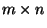-matrix A and an m-vector b, specifying a regionby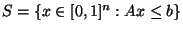.

• SOLUTION: A multivariate polynomial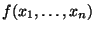of total degree at most 2.

• MEASURE: The maximum value of f in the region specified by the linear constants, i.e.,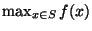.

• Bad News: Does not admit a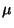-approximation for any constant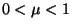.

• Comment: A-approximation algorithm finds a solution that differs from the optimal solution by at most the value. Variation in which we look for a polynomial f of any degree does not admit a-approximation forfor some. Note that these problems are known to be solvable in polynomial space but are not known to be in NP.

• Garey and Johnson: MP2

Viggo Kann
2000-03-20